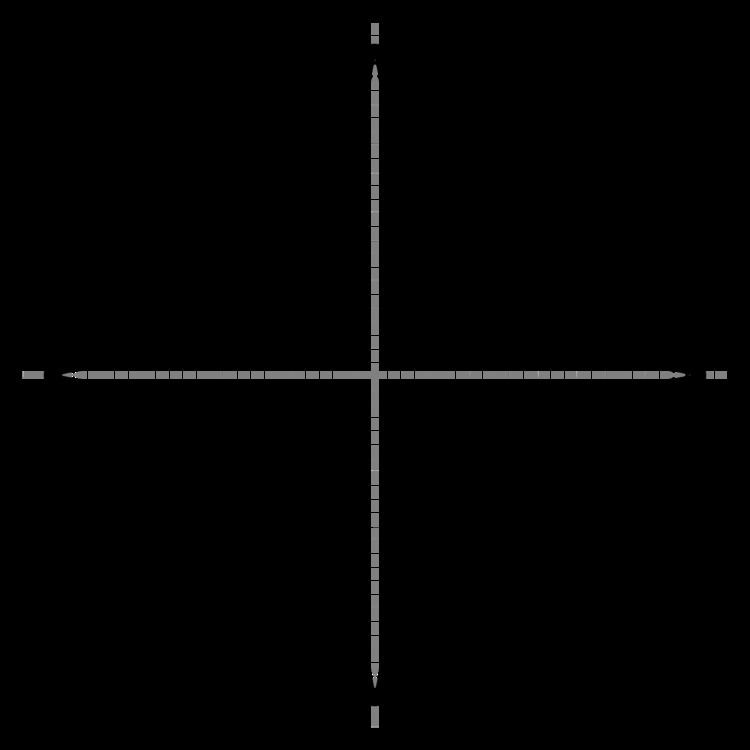# Astroid

Updated onAn astroid is a particular mathematical curve: a hypocycloid with four cusps. Specifically, it is the locus of a point on a circle as it rolls inside a fixed circle with four times the radius. By double generation, it is also the locus of a point on a circle as it rolls inside a fixed circle with 4/3 times the radius. It can also be defined as the envelope of a line segment with an end point on each of the axes. It is therefore the envelope of the moving bar in the Trammel of Archimedes.

## Contents

Its modern name comes from the Greek word for "star". The curve had a variety of names, including tetracuspid (still used), cubocycloid, and paracycle. It is nearly identical in form to the evolute of an ellipse.

## Equations

If the radius of the fixed circle is a then the equation is given by

x 2 / 3 + y 2 / 3 = a 2 / 3 .

This implies that an astroid is also a superellipse.

Parametric equations are

x = a cos 3 t = a 4 ( 3 cos t + cos 3 t ) , y = a sin 3 t = a 4 ( 3 sin t sin 3 t ) .

The pedal equation with respect to the origin is

r 2 = a 2 3 p 2 ,

the Whewell equation is

s = 3 a 4 cos 2 φ ,

and the Cesàro equation is

R 2 + 4 s 2 = 9 a 2 4 .

The polar equation is

r = a ( cos 2 / 3 θ + sin 2 / 3 θ ) 3 / 2 .

The astroid is a real locus of a plane algebraic curve of genus zero. It has the equation

( x 2 + y 2 a 2 ) 3 + 27 a 2 x 2 y 2 = 0.

The astroid is therefore a real algebraic curve of degree six.

## Metric properties

Area enclosed
3 8 π a 2
Length of curve
6 a
Volume of the surface of revolution of the enclose area about the x-axis.
32 105 π a 3
Area of surface of revolution about the x-axis
12 5 π a 2

## Properties

The astroid has four cusp singularities in the real plane, the points on the star. It has two more complex cusp singularities at infinity, and four complex double points, for a total of ten singularities.

The dual curve to the astroid is the cruciform curve with equation x 2 y 2 = x 2 + y 2 . The evolute of an astroid is an astroid twice as large.

Similar Topics
Astroid Boys
Astroides
Tia Ballard
Topics